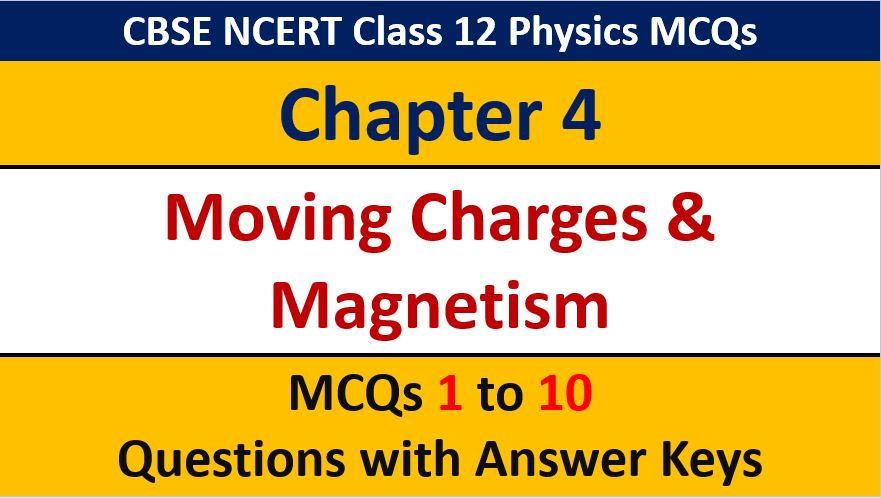## MCQ Questions for Class 12 Physics Chapter 4 Moving charges and Magnetism with Answer Keys

If you are preparing for CBSE Class 12 Board Exam Term 1 which will be an objective type questions paper, then you need to practice multiple choice questions of Class 12 Physics Chapterwise. In this article of AKVTutorials, you will get MCQ Questions for Class 12 Physics Chapter 4 Moving charges and Magnetism with Answer Keys.

MCQ Questions for Class 12 Physics Chapter 4 Question No 1:

Magnetic lines of force due to a straight conductor carrying current are

Option A : Straight lines

Option B : Elliptical

Option C : Circular

Option D : Parabolic

Option C : Circular

MCQ Questions for Class 12 Physics Chapter 4 Question No 2:

Magnetic field at a point on the line of current carrying conductor is

Option A : Maximum

Option B : Infinity

Option C : Zero

Option D : Finite value

Option C : Zero

MCQ Questions for Class 12 Physics Chapter 4 Question No 3:

Among the following are is incorrect in the case of straight current carrying conductor

Option A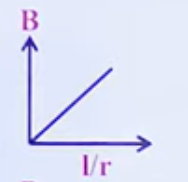Option B :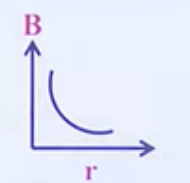Option C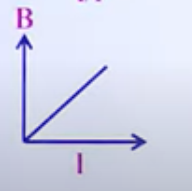Option D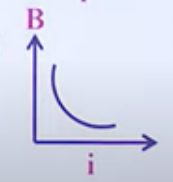Option D :

MCQ Questions for Class 12 Physics Chapter 4 Question No 4:

The figure shows a uniform magnetic field B directed to the left and a wire carrying a current into the page. The magnetic force acting on the wire is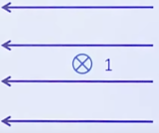Option A : Towards the top of the page

Option B : Towards the bottom of the page

Option C : Towards the left

Option D : Towards the right

Option A : Towards the top of the page

MCQ Questions for Class 12 Physics Chapter 4 Question No 5:

For a given distance from a current element the magnetic induction is maximum at an angle measured with respect to axis of the current

Option A : 3π /4

Option B : π/4

Option C :  π/2

Option D : 2/π

Option C : π/2

MCQ Questions for Class 12 Physics Chapter 4 Question No 6:

A uniform magnetic field is directed into the page. A charged particle, moving in the plane of the page, follows a clockwise spiral of decreasing radius as shown. A reasonable explanation is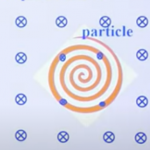Option A : The charge is positive and slowing down

Option B : The charge is negative and slowing down

Option C : The charge is positive and speeding up

Option D : The charge is negative and speeding up

Option B : The charge is negative and slowing down

MCQ Questions for Class 12 Physics Chapter 4 Question No 7:

A circular coil of wire carries a current. PQ is a part of very long wire carrying a current and passing close to the circular coil, if the directions of currents are those shown in figure, then the direction of the force acting of PQ is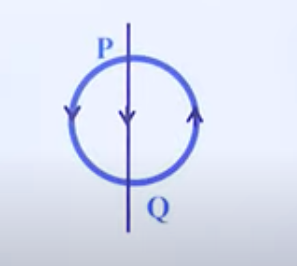Option A : Parallel to PQ, towards p

Option B : Parallel to PQ, towards Q

Option C : At right angles to PQ, to the right

Option D : At right angles to PQ, to the left

Option D : At right angles to PQ, to the left

MCQ Questions for Class 12 Physics Chapter 4 Question No 8:

A  circular coil has one turn and carries a current ‘i’. The same wire is wound into a smaller coil of 4 turns and the same current is passed through it. Field at the center

Option A : Decreases to 1/4 of the value

Option B : Is the same

Option C : Increases to 14 times the value

Option D : Increases to 16 times the value

Option D : Increases to 16 times the value

MCQ Questions for Class 12 Physics Chapter 4 Question No 9:

A rectangular loop carrying current i is located near an infinite loop straight conductor carrying current i is a shown in the figure, The loop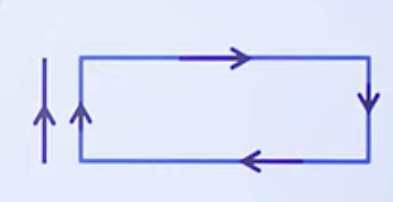Option A : Remains stationary

Option B : Is attracted towards the wire

Option C : Is repelled away from the wire

Option D : Will rotate about an axis parallel to the wire

Option B : Is attracted towards the wire

MCQ Questions for Class 12 Physics Chapter 4 Question No 10:

A wire of length 44cm is bent into a circle and a current of 9.8A is passed through it. The intensity of magnetic induction at the centre of the circle is

Option A : 8.8 × 10-6 T

Option B : 8.8 × 10-5 T

Option C : 8.4 × 10-6 T

Option D : 6.1 × 10-5 T

Option B : 8.8 × 10-5 T

CBSE Class 12 Physics Chapterwise MCQ
1 : Electric Charge & Fields MCQs
| 1 to 10 | 11 to 20
2 : Electrostatics Potential and Capacitance MCQs
| 1 to 10 | 11 to 20
3 : Current Electricity MCQs
| 1 to 10 | 11 to 20
4 : Moving charges and Magnetism MCQs
| 1 to 10 | 11 to 20
5 : Magnetism and Matter MCQs
| 1 to 10 | 11 to 20
6 : Electromagnetic Induction MCQs
| 1 to 10 | 11 to 20
7 : Alternating Current MCQs
| 1 to 10 | 11 to 20
8 : Electromagnetic Waves MCQs
| 1 to 10 | 11 to 20
9 : Ray Optics and Optical Instruments MCQs
| 1 to 10 | 11 to 20
10 : Wave Optics MCQs
| 1 to 10 | 11 to 20
11 : Dual Nature of Radiation MCQs
| 1 to 10 | 11 to 20
12 : Atoms MCQs
| 1 to 10 | 11 to 20
13 : Nuclei MCQs
| 1 to 10 | 11 to 20
14 : Semi Conductor Electronics MCQs
| 1 to 10 | 11 to 20
15 : Communication Systems MCQs
| 1 to 10 | 11 to 20